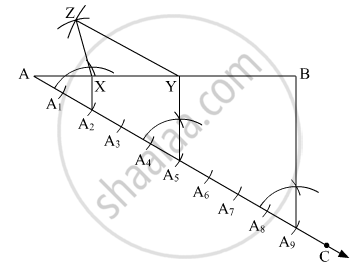Advertisement Remove all ads

# The Perimeter of a Triangle is 14.4 Cm and the Ratio of Lengths of Its Side is 2 : 3 : 4. Construct the Triangle. - Geometry

Diagram

The perimeter of a triangle is 14.4 cm and the ratio of lengths of its side is 2 : 3 : 4. Construct the triangle.

Advertisement Remove all ads

#### SolutionSteps of construction:
1: A line AB = 14.4 cm is drawn.

2: Darw a ray AC making an acute angle at A in the downward direction. Then, divid it into (2 + 3 + 4) = 9 parts.

3: Join B and A9.

4:  Draw a line parallel to BA9 at A2 and A5 intersecting AB at X and Y respectively..

5: With X as centre and XA as radius, draw an arc.

6: With Y as centre and YB as radius, draw an arc cutting the previous arc at Z.

7: Join XZ and YZ.

Hence, △XYZ is the required triangle.

Is there an error in this question or solution?
Advertisement Remove all ads

#### APPEARS IN

Balbharati Mathematics 2 Geometry 9th Standard Maharashtra State Board
Chapter 4 Constructions of Triangles
Problem Set 4 | Q 3 | Page 56
Advertisement Remove all ads
Advertisement Remove all ads
Share
Notifications

View all notifications

Forgot password?